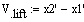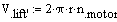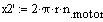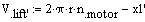# Question about a dynamic equation of a crane model

Iqbal94
Hi guys,

I have a question on the fundamental dynamic equation as I am not sure if my equation is correct. I am doing an analysis on a portal crane to find its application factor, somehow I couldn't get the desired value for the velocity of each masses as I couldn't find the tension of the rope. The portal crane model is simplified to be (picture).

http://[ATTACH=full]200070[/ATTACH]

#### Attachments

Last edited by a moderator:

## Answers and Replies

OldYat47
Since it's a dynamic situation you have to get an idea of the frequency of oscillation at the spring and the rope separately (two sine waves). Probably the two won't be in sync so there will be constructive and destructive interaction. So the load in both the spring and the rope will change over time. There are available equations for doing this calculation. The static tension in the rope is ML X G. The static tension in the spring is the sum of the masses X G. When you move the crane the tensions will increase (acceleration of the loads, which you'll have to know), then the "springiness" will start the loads oscillating.

Your sketch isn't very clear. Is the crane lifting the load, translating the load, or both?

•Iqbal94
Iqbal94
Since it's a dynamic situation you have to get an idea of the frequency of oscillation at the spring and the rope separately (two sine waves). Probably the two won't be in sync so there will be constructive and destructive interaction. So the load in both the spring and the rope will change over time. There are available equations for doing this calculation. The static tension in the rope is ML X G. The static tension in the spring is the sum of the masses X G. When you move the crane the tensions will increase (acceleration of the loads, which you'll have to know), then the "springiness" will start the loads oscillating.

Your sketch isn't very clear. Is the crane lifting the load, translating the load, or both?
The crane is lifting the load. Yes, I have the frequency of oscillation at the spring. From the tests and analysis that I've done, I found out that there is only 1 frequency peek which is for the spring. I used steel rope/wire during the tests and I assume due to its rigidity, the frequency due to load on the spring can be neglected. Anyway, I would like to confirm either the lifting velocity of the crane is equal to the difference of velocity of load(x'load) and crane body(x'm1)or#### Attachments

OldYat47
The tension on a reasonably "rigid" rope would be equal to ML X G. You have the velocity of the lift correct. That's at steady lifting speed. If the load starts out static then there's addition tension in the wire rope due to acceleration of the load. There will be a fluctuating component equal to the oscillation rate and magnitude of the spring. That's because the load accelerates and decelerates with the extension and retraction of the spring.

Iqbal94
Hi, thanks for your explanation. I have one more question, is it possible to know when the spring will start to oscillate? Is it right after the load is lifted, or is there any circumstance that I need to take note? I'm not sure if my idea is right. But I think the spring starts to oscillate (the load as well), when the rope tension is equal to the initial spring force?